## Electrostatics

Electrostatics, as the name implies, is the study of stationary electric charges. A rod of plastic rubbed with fur or a rod of glass rubbed with silk will attract small pieces of paper and is said to be electrically charged. The charge on plastic rubbed with fur is defined as negative, and the charge on glass rubbed with silk is defined as positive.

## Electric charge

Electrically charged objects have several important characteristics:

• Like charges repel one another; that is, positive repels positive and negative repels negative.
• Unlike charges attract each another; that is, positive attracts negative.
• Charge is conserved. A neutral object has no net charge. If the plastic rod and fur are initially neutral, when the rod becomes charged by the fur, a negative charge is transferred from the fur to the rod. The net negative charge on the rod is equal to the net positive charge on the fur.

A conductor is a material through which electric charges can easily flow. An insulator is a material through which electric charges do not move easily, if at all. An electroscope is a simple device used to indicate the existence of charge. As shown in Figure 1, the electroscope consists of a conducting knob and attached lightweight conducting leaves—commonly made of gold foil or aluminum foil. When a charged object touches the knob, the like charges repel and force the leaves apart. The electroscope will indicate the presence of charge but does not directly indicate whether the charge is positive or negative.Figure 1

An electroscope reports the presence of charge.

A large charge near a neutral electroscope can make the leaves move apart. The electroscope is made of conducting material, so the positive charges are attracted to the knob by the nearby (but not touching) negatively charged rod. The leaves are left with a negative charge and therefore deflect. When the negative rod is removed, the leaves will fall.

Now, consider touching the electroscope knob with a finger while the charged rod is nearby. The electrons will be repulsed and flow out of the electroscope through the hand. If the hand is removed while the charged rod is still close, the electroscope will retain a charge. This method of charging is called charging by induction (see Figure 2).Figure 2

Charging an electroscope by induction.

When an object is rubbed with a charged rod, the object shares the charge so that both have a charge of the same sign. In contrast, charging by induction gives an object the charge opposite that of the charged rod.

Even though the charges are not free to travel throughout the material, insulators can be charged by induction. A large charge nearby—not touching—will induce an opposite charge on the surface of the insulator. As shown in Figure 3, the negative and positive charges of the molecules are displaced slightly. This realignment of charges in the insulator produces an effective induced charge.Figure 3

Induction of surface charge on an insulator by a nearby charged object.

## Coulomb's law

Coulomb's law gives the magnitude of the electrostatic force ( F) between two charges: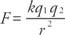where q 1 and q 2 are the charges, r is the distance between them, and k is the proportionality constant. The SI unit for charge is the coulomb. If the charge is in coulombs and the separation in meters, the following approximate value for k will give the force in newtons: k = 9.0 × 10 9 N · m 2/C 2. The direction of the electrostatic force depends upon the signs of the charges. Like charges repel, and unlike charges attract.

Coulomb's law can also be expressed in terms of another constant (ε 0), known as the permittivity of free space:When the permittivity constant is used, Coulomb's law is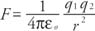The most fundamental electric charge is the charge of one proton or one electron. This value (e) is e = 1.602 × 10 −19 coulombs. It takes about 6.24 × 10 18 excess electrons to equal the charge of one coulomb; thus, it is a very large static charge.

For a system of charges, the forces between each set of charges must be found; then, the net force on a given charge is the vector sum of these forces. The following problem illustrates this procedure.

Example 1: Consider equal charges of Q whose value in coulombs is not known. The force between two of these charges at distance X is F. In Figure , three charges (3 Q) are placed at point A, which is a distance X from point B. One charge (Q) is placed at point B, which is X/2 distance from point C, which has one charge. What is the net force on the charge at point B?Figure 4

Arrangement of point charges for the example.

Solution: This problem can be solved through proportional reasoning. The force of 3 Q on the one charge at B will be 3 F. Because the single charge is one‐half X from B, the force will be four times greater than at a distance X, that is, 4 F. The forces of 3 F and 4 F are at right angles, and therefore, the resultant force is 5 F, orThe direction is found from the tangent: θ −1 = tan 4/3 = 53°

## Electric fields and lines of force

When a small positive test charge is brought near a large positive charge, it experiences a force directed away from the large charge. If the test charge is far from the large charge, the electrostatic force given by Coulomb's law is smaller than when it is near. This data of direction and magnitude of an electrostatic force, due to a fixed charge or set of fixed charges, constitutes an electrostatic field. The electric field is defined as the force per unit charge exerted on a small positive test charge (q 0) placed at that point. Mathematically,Note that both the force and electric field are vector quantities. The test charge is required to be small so that the field of the test charge does not affect the field of the set charges being examined. The SI unit for electric field is newtons per coulomb (N/C).

Figure 5 is a pictorial representation of the electric fields surrounding a positive charge and a negative charge. These lines are called field lines or lines of force.Figure 5

Electric field lines of (a) positive and (b) negative point charges.

Figure 6 shows the electric fields for opposite charges, similar charges, and oppositely charged plates.Figure 6

Electric field lines of (a) two opposite charges, (b) two like charges, and (c) two oppositely charged plates.

The rules for drawing electric field lines for any static configuration of charges are

• The lines begin on positive charges and terminate on negative charges.
• The number of lines drawn emerging from or terminating on a charge is proportional to the magnitude of the charge.
• No two field lines ever cross in a charge‐free region. (Because the tangent to the field line represents the direction of the resultant force, only one line can be at every point.)
• The line approaches the conducting surface perpendicularly.

Electric flux

Electric flux is defined as the number of field lines that pass through a given surface. In Figure , lines of electric flux emerging from a point charge pass through an imaginary spherical surface with the charge at its center.Figure 7

Electric flux due to a point charge + q.

This definition can be expressed as follows: Φ = ∑E · A, where Φ (the Greek letter phi) is the electric flux, E is the electric field, and A is area perpendicular to the field lines. Electric flux is measured in N · m 2 / C 2 and is a scalar quantity. If the surface under consideration is not perpendicular to the field lines, then the expression is Φ = ∑ EA cos θ.

In general terms, flux is the closed integral of the dot product of the electric field vector and the vector ΔA. The direction of ΔA is the outward drawn normal to the imaginary surface. Mathematically, Φ = ΦE · dA. The accepted convention is that flux lines are positive if leaving a surface and negative if entering a surface.

## Gauss's law

Gauss's law provides a method to calculate any electric field; however, its only practical use is for fields of highly symmetric distributions of fixed charges. The law states that the net electric flux through any real or imaginary closed surface is equal to the net electric charge enclosed within that surface divided by ε. As a result, if no charge exists with a given closed surface, then there are as many flux lines entering the surface as there are leaving it. The imaginary surface necessary to apply Gauss's law is called the gaussian surface. Algebraically,or in integral form,where θ is the angle between the direction of E and the outward direction of normal to the surface and ε is the permittivity constant.

Consider the calculation of the electric field due to a point charge. Figure shows the point charge, the direction of its field, and a gaussian surface. Because the electric field is perpendicular to the gaussian surface and directed outward, θ is 90 degrees, and cos θ = 1. Gauss's law is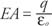Substitute in the area of a sphere, and the left side reduces toor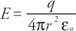which is the same expression obtained from Coulomb's law and the definition of electric field in terms of force.

The derivation of the expression for the field due to a thin conducting shell of charge follows. Figure 8 shows the electric fields for (a) a shell of radius ( R), (b) the gaussian surface for outside the shell, and (c) the gaussian surface for inside the shell (c) of radius ( r).Figure 8

A charged spherical shell of radius R. (b) A gaussian sphere with radius r >R. (c) A gaussian sphere with radius r < R.

When outside the shell of charge, as in Figure (a), the left side of Gauss's equation reduces to the following expression for the same reasons given for a point charge: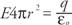Therefore,Thus, the electric field outside a sphere of charge is the same as if the same amount of charge were concentrated in a point located at the center of the sphere.

The gaussian surface inside the sphere encloses no charge, and therefore, there is no electric field inside the uniformly charged spherical shell. The same proof holds within a solid conductor because all the charge of the conductor resides on the surface. Because the electric field inside even an irregularly shaped conductor is zero, the charge will not be evenly distributed over an irregular shape. The charge will tend to accumulate on protruding points on the outside of the conductor.

## Potential difference and equipotential surfaces

In the preceding examples, the charge distributions were spherical, and so the gaussian surface was a sphere. When finding the electric field of either a sheet of charge or a line of charge, a cylinder is the correct gaussian surface to use.

Example 2: Find the electric field for a nonconducting infinite sheet of charge. The electric field is directed outward from the sheet on both sides. The charge per unit area is σ (the Greek letter sigma). See Figure for the electric field and gaussian surface.Figure 9

A gaussian cylinder must extend above and below an infinite sheet of charge.

The area of the closed cylindrical gaussian surface will be the sum of the areas of the left end, the right end, and the wall; therefore, ∑ EA cos θ = ( EA cos θ) left end + ( EA cos θ) right end + ( EA cos θ) wall. The electric field is parallel to the wall, which is at right angles to the outward normal of the wall area; thus, the last term on the right is zero. At each end, E is in the same direction as the outward normal, so ( EA cos θ) left end + ( EA cos θ) right end = 2 EA, where A is the area of the end of the gaussian cylinder. The total charge inside the gaussian surface is the product of the charge per unit area and the area; soand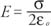Note that the magnitude of the electric field does not depend upon the distance from the plate. The electric field is uniform. In the practical case of finite plates of charge, the electric field is uniform relatively close to the charged plate.

The resultant electric field of two parallel plates is double that of one sheet with the same charge: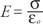or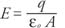where q is the charge on each plate, and A is the area of each plate. If the plates have opposite charges, the electric field will exist between the plates and be zero outside the plates. If the charges are of equal sign, the electric field will be zero between the plates and be expressed by the above equation outside the plates. These results can be derived by Gauss's law.

## Electrostatic potential and equipotential surfaces

Imagine moving a small test charge q′ from point A to point B in the uniform field between parallel plates. The work done in transferring the charge equals the product of the force on the test charge and the parallel component of displacement, using the same definition of work given in the section on mechanics. This work can also be expressed in terms of E from the definition of electric field as the ratio of force to charge: W · d, E = F/ q and W = q′. See Figure 10.Figure 10

Work is done when q′ moves from position A to B in an electric fieldE.

Work is change in potential energy: U B U A = qEd.

In general, the electrostatic potential difference, sometimes called the electric potential difference, is defined as the energy change per unit positive charge, or V B V A = ( U B U A )/ q′. For certain configurations of electric field, it may be necessary to use the integral definition of electrostatic potential:where a test charge moves over a line integral from point A to point B along path s in an electric field ( E).

For the special case of parallel plates:where V is the potential difference between the plates, measured in units of volts (V):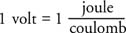The electric potential due to a point charge (q) at a distance (r) from the point charge is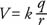The following problem illustrates the calculations of electric field and potential due to point charges.

Example 3: Given two charges of +3 Q and – Q, a distance X apart, find the following: (1) At what point(s) along the line is the electric field zero? (2) At what point(s) is the electric potential zero? (See Figure 11.)Figure 11

The arrangement of two point charges for the example.

The first task is to find the region(s) where the electric field is zero. The electric field is a vector, and its direction can be located by a test charge. Figure is divided into three regions. Between the opposite charges, the direction of the force on the test charge will be in the same direction from each charge; therefore, it is impossible to have a zero electric field in Region II. Even though the forces on the test charge from the two charges are in opposite directions in Region I, the force, and therefore the electric field, can never be zero in this region because the test charge is always closer to the largest given charge. Therefore, Region III is the only place where E can be zero. Select an arbitrary point (r) to the right of – Q and set the two electric fields equal. Because the fields are in opposite directions, the vector sum at this point will equal zero.If X is given, solve for r.

Potential is not a vector, so the potential is zero wherever the following equation holds: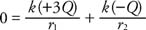where r m is the distance from the test point to +3 Q and r 2 is the distance to– Q.

This example illustrates the difference in methods of analysis in finding the vector quantity ( E) and the scalar quantity (V). Note that if the charges were either both positive or both negative, it would be possible to find a point with zero electric field between the charges, but the potential would never be zero.

The electrical potential energy of a pair of point charges separated by a distance r isEquipotential surfaces are surfaces where no work is required to move a charge from one point to another. The equipotential surfaces are always perpendicular to the electric field lines. Equipotential lines are two‐dimensional representations of the intersection of the surface with the plane of the diagram. In Figure , equipotential lines are shown for (a) a uniform field, (b) a point charge, and (c) two opposite charges.Figure 12

Equipotential lines for (a) a uniform electric field, (b) a point charge, and (c) a dipole.